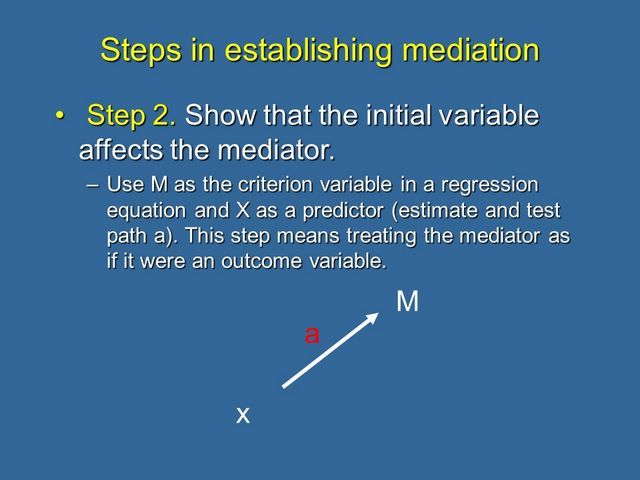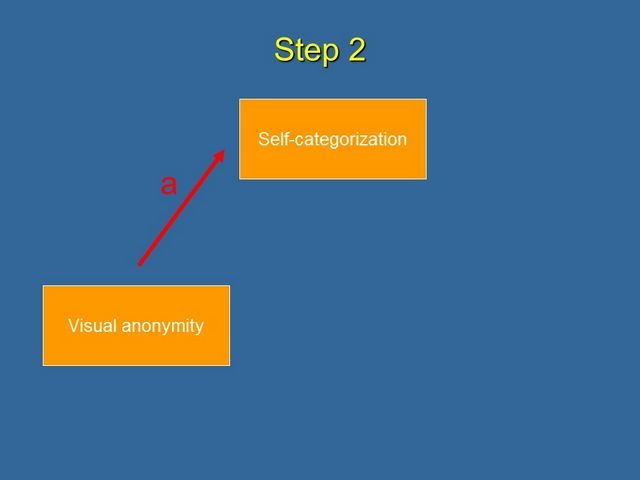# 8. Causal Steps to Establish Mediation: Step 2In the second step, we need to show that the initial, or predictor, variable affects the mediator. So we perform another simple linear regression using the mediator as if it were the outcome variable and regressing it on the predictor, which gives us an estimate of path a.

In our example, path a describes the effect of Visual Anonymity (predictor) on Self-categorization (mediator).9. Causal Steps to Establish Mediation: Steps 3 and 4